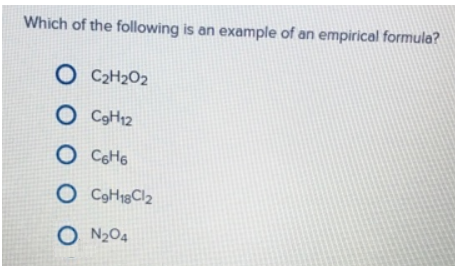# Problem: Which of the following is an example of an empirical formula? (a) C2H2O2 (b) C9H12 (c) C6H6(d) C9H18Cl2(e) N2O4

###### FREE Expert Solution

Empirical formula must be in the least common denominator for the subscript.

(a) C2H2O2 (can be simplified as CHO)

93% (34 ratings)###### Problem Details

Which of the following is an example of an empirical formula?

(a) C2H2O2

(b) C9H12

(c) C6H6

(d) C9H18Cl2

(e) N2O4Frequently Asked Questions

What scientific concept do you need to know in order to solve this problem?

Our tutors have indicated that to solve this problem you will need to apply the Empirical Formula concept. You can view video lessons to learn Empirical Formula. Or if you need more Empirical Formula practice, you can also practice Empirical Formula practice problems.

What professor is this problem relevant for?

Based on our data, we think this problem is relevant for Professor Zellmer's class at OSU.